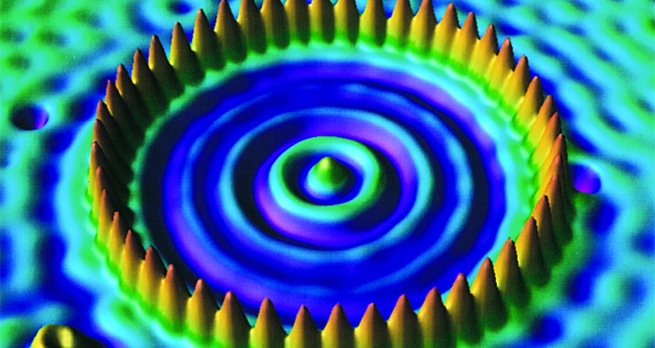Collisions and conservation laws

Start this free course now. Just create an account and sign in. Enrol and complete the course for a free statement of participation or digital badge if available.

Free course

# Glossary

collision
A brief interaction between two or more particles or bodies in close proximity.
completely inelastic collision
A collision in which the colliding bodies stick together, resulting in the maximum loss of kinetic energy consistent with conservation of momentum.
conservation of kinetic energy
The principle that the total kinetic energy of any isolated system is constant.
conservation of linear momentum
The principle that the total linear momentum of any isolated system is constant.
elastic collision
A collision in which kinetic energy is conserved.
inelastic collision
A collision in which kinetic energy is not conserved.
internal force
In the context of a given system, an internal force is a force that acts within the system and which has a reaction that also acts within the system.
isolated system
A system which cannot exchange matter or energy with its environment. In the context of mechanics, an isolated system is one that is subject only to internal forces.
kinetic energy
The energy that a body possesses by virtue of its motion.
law of conservation of linear momentum
See conservation of linear momentum.
linear momentum
The momentum associated with the translational motion of a body. For a particle of mass travelling with velocity , the linear momentum is .
mass energy
The energy that a body possesses by virtue of its mass, as given by , where is the speed of light in a vacuum. The existence of mass energy is one of the many implications of the special theory of relativity. The mass energy of a free particle is the difference between its (total) relativistic energy and its relativistic translational kinetic energy. Mass energy is also known as rest energy.
momentum
A vector quantity, useful in various situations as a measure of a body's tendency to continue in its existing state of rotational or translational motion.
principle of conservation of linear momentum
See conservation of linear momentum.
relativistic collision
A collision involving sufficiently high speeds that its analysis requires the use of the relativistic relations for momentum and energy rather than their Newtonian counterparts. Relativistic collisions are often inelastic and are characterised by the creation of new particles and an associated increase in mass energy (at the expense of kinetic energy).
relativistic energy
According to the theory of special relativity the total energy (including the mass energy) of a particle of mass travelling with speed is
relativistic kinetic energy
According to the theory of special relativity, the translational kinetic energy of a particle of mass and speed is equal to its total relativistic energy minus its mass energy
relativistic momentum
The momentum of a body according to the special theory of relativity. For a particle of (rest) mass , travelling with velocity , the relativistic momentum is At speeds which are small compared with the speed of light, , this reduces to the Newtonian expression .
superelastic collision
A collision in which the kinetic energy increases, typically as a result of the release of potential energy.
system
That part of the Universe which is the subject of an investigation.
S217_1